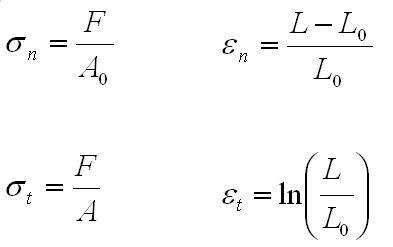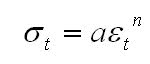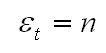# Calculating Nominal strain at which Necking Occurs

• 50Cent

## Homework Statement

Given two wires of gauge length 100mm and 200mm
They are loaded to fracture in tension
The 100mm sample extends by 15mm
The 200mm sample extends by 25mm.

I need to use this information to calculate the nominal strain at which necking commences in the wires

## Homework Equations

These are the relavent equations i can find in my notes relating to necking, stress/strain, true stress/strainwe are also told, the true stress/strain curve generally represented by,where n is the work-hardening exponent

"necking" in a sample started when the value of the true strain is equal to the work hardening exponent, i.e.,## The Attempt at a Solution

Im not so sure where to start with this as there are no forces given or areas. I assume we have to use the two sets of data to equated these terms and cancel them out.